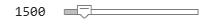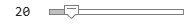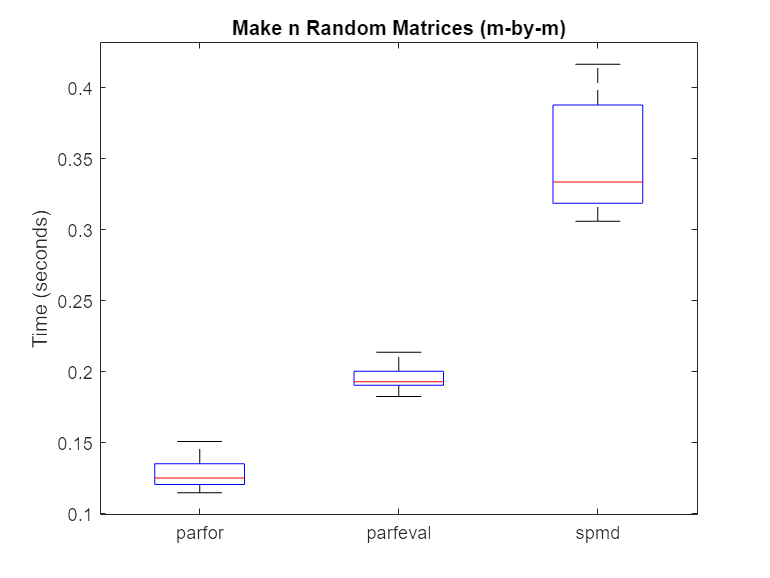Choose Between spmd, parfor, and parfeval

Communicating Parallel Code

To run computations in parallel, you can use parfor, parfeval, parfevalOnAll, or spmd. Each construct relies on different parallel programming concepts. If you require workers to communicate throughout a computation, use parfeval, parfevalOnAll, or spmd.

• Use parfeval or parfevalOnAll if your code can be split into a set of tasks, where each task can depend on the output of other tasks.

• Use spmd if you require communication between workers during a computation.

Computations with parfeval are best represented as a graph, similar to a Kanban board with blocking. Generally, results are collected from workers after a computation is complete. You can collect results from execution of a parfeval operation by using afterEach or afterAll. You typically use the results in further calculations.

Computations with spmd are best represented by a flowchart, similar to a waterfall workflow. A pool worker executing spmd statements is called a lab. Results can be collected from labs during a computation. Sometimes, labs must communicate with other labs before they can finish their computation.

If you are unsure, ask yourself the following: within my communicating parallel code, can each computation be completed without any communication between workers? If yes, use parfeval. Otherwise, use spmd.

Synchronous and Asynchronous Work

When choosing between parfor, parfeval, and spmd, consider whether your calculation requires synchronization with the client.

parfor and spmd require synchronization, and therefore block you from running any new computations on the MATLAB® client. parfeval does not require synchronization, so the client is free to pursue other work.

Compare Performance of Multithreading and ProcessPool

In this example, you compare how fast functions run on the client and on a ProcessPool. Some MATLAB functions make use of multithreading. Tasks that use these functions perform better on multiple threads than a single thread. Therefore, if you use these functions on a machine with many cores, a local cluster can perform worse than multithreading on the client.

The supporting function clientFasterThanPool, listed at the end of this example, returns true if multiple executions are performed faster on the client than a parfor-loop. The syntax is the same as parfeval: use a function handle as the first argument, the number of outputs as the second argument, and then give all required arguments for the function.

First, create a local ProcessPool.

p = parpool('local');
Starting parallel pool (parpool) using the 'local' profile ...
Connected to the parallel pool (number of workers: 6).

Check how fast the eig function runs by using the clientFasterThanPool supporting function. Create an anonymous function with eig to represent your function call.

[~, t_client, t_pool] = clientFasterThanPool(@(N) eig(randn(N)), 0, 500)
t_client = 22.6243
t_pool = 4.9334

The parallel pool computes the answer faster than the client. Divide t_client by maxNumCompThreads to find the time taken per thread on the client.

ans = 3.7707

Workers are single threaded by default. The result indicates that the time taken per thread is similar on both the client and the pool, as the value of t_pool is roughly 1.5 times the value of t_client/maxNumCompThreads. The eig function does not benefit from multithreading.

Next, check how fast the lu function runs by using the clientFasterThanPool supporting function.

[~, t_client, t_pool] = clientFasterThanPool(@(N) lu(randn(N)), 0, 500)
t_client = 1.0225
t_pool = 0.4693

The parallel pool typically computes the answer faster than the client if your local machine has four or more cores. Divide t_client by maxNumCompThreads to find the time taken per thread.

ans = 0.1704

This result indicates that the time taken per thread is much less on the client than the pool, as the value of t_pool is roughly 3 times the value of t_client/maxNumCompThreads. Each thread is used for less computational time, indicating that lu uses multithreading.

Define Helper Function

The supporting function clientFasterThanPool checks whether a computation is faster on the client than on a parallel pool. It takes as input a function handle fcn and a variable number of input arguments (in1, in2, ...). clientFasterThanPool executes fcn(in1, in2, ...) on both the client and the active parallel pool. As an example, if you wish to test rand(500), your function handle must be in the following form:

fcn = @(N) rand(N);

Then, use clientFasterThanPool(fcn,500).

function [result, t_multi, t_single] = clientFasterThanPool(fcn,numout,varargin)
% Preallocate cell array for outputs
outputs = cell(numout);

% Client
tic
for i = 1:200
if numout == 0
fcn(varargin{:});
else
[outputs{1:numout}] = fcn(varargin{:});
end
end
t_multi = toc;

% Parallel pool
vararginC = parallel.pool.Constant(varargin);
tic
parfor i = 1:200
% Preallocate cell array for outputs
outputs = cell(numout);

if numout == 0
fcn(vararginC.Value{:});
else
[outputs{1:numout}] = fcn(vararginC.Value{:});
end
end
t_single = toc;

% If multhreading is quicker, return true
result = t_single > t_multi;
end

Compare Performance of parfor, parfeval, and spmd

Using spmd can be slower or faster than using parfor-loops or parfeval, depending on the type of computation. Overhead affects the relative performance of parfor-loops, parfeval, and spmd.

For a set of tasks, parfor and parfeval typically perform better than spmd under these conditions.

• The computational time taken per task is not deterministic.

• The computational time taken per task is not uniform.

• The data returned from each task is small.

Use parfeval when:

• You want to run computations in the background.

In this example, you examine the speed at which matrix operations can be performed when using a parfor-loop, parfeval, and spmd.

First, create a local parallel pool p.

p = parpool('local');
Starting parallel pool (parpool) using the 'local' profile ...
Connected to the parallel pool (number of workers: 6).

Compute Random Matrices

Examine the speed at which random matrices can be generated by using a parfor-loop, parfeval, and spmd. Set the number of trials (n) and the matrix size (for an m-by-m matrix). Increasing the number of trials improves the statistics used in later analysis, but does not affect the calculation itself.

m =1000;
n =20;

Then, use a parfor-loop to execute rand(m) once for each worker. Time each of the n trials.

parforTime = zeros(n,1);
for i = 1:n
tic;
mats = cell(1,p.NumWorkers);
parfor N = 1:p.NumWorkers
mats{N} = rand(m);
end
parforTime(i) = toc;
end

Next, use parfeval to execute rand(m) once for each worker. Time each of the n trials.

parfevalTime = zeros(n,1);
for i = 1:n
tic;
f(1:p.NumWorkers) = parallel.FevalFuture;
for N = 1:p.NumWorkers
f(N) = parfeval(@rand,1,m);
end
mats = fetchOutputs(f, "UniformOutput", false)';
parfevalTime(i) = toc;
clear f
end

Finally, use spmd to execute rand(m) once for each lab. For details on labs and how to execute commands on them with spmd, see Run Single Programs on Multiple Data Sets. Time each of the n trials.

spmdTime = zeros(n,1);
for i = 1:n
tic;
spmd
e = rand(m);
end
eigenvals = {e{:}};
spmdTime(i) = toc;
end

Use rmoutliers to remove the outliers from each of the trials. Then, use boxplot to compare the times.

% Hide outliers
boxData = rmoutliers([parforTime parfevalTime spmdTime]);

% Plot data
boxplot(boxData, 'labels',{'parfor','parfeval','spmd'}, 'Symbol','')
ylabel('Time (seconds)')
title('Make n random matrices (m by m)')Typically, spmd requires more overhead per evaluation than parfor or parfeval. Therefore, in this case, using a parfor-loop or parfeval is more efficient.

Compute Sum of Random Matrices

Next, compute the sum of random matrices. You can do this by using a reduction variable with a parfor-loop, a sum after computations with parfeval, or gplus with spmd. Again, set the number of trials (n) and the matrix size (for an m-by-m matrix).

m =1000;
n =20;

Then, use a parfor-loop to execute rand(m) once for each worker. Compute the sum with a reduction variable. Time each of the n trials.

parforTime = zeros(n,1);
for i = 1:n
tic;
result = 0;
parfor N = 1:p.NumWorkers
result = result + rand(m);
end
parforTime(i) = toc;
end

Next, use parfeval to execute rand(m) once for each worker. Use fetchOutputs on all of the matrices, then use sum. Time each of the n trials.

parfevalTime = zeros(n,1);
for i = 1:n
tic;
f(1:p.NumWorkers) = parallel.FevalFuture;
for N = 1:p.NumWorkers
f(N) = parfeval(@rand,1,m);
end
result = sum(fetchOutputs(f));
parfevalTime(i) = toc;
clear f
end

Finally, use spmd to execute rand(m) once for each lab. Use gplus to sum all of the matrices. To send the result only to the first lab, set the optional targetlab argument to 1. Time each of the n trials.

spmdTime = zeros(n,1);
for i = 1:n
tic;
spmd
r = gplus(rand(m), 1);
end
result = r{1};
spmdTime(i) = toc;
end

Use rmoutliers to remove the outliers from each of the trials. Then, use boxplot to compare the times.

% Hide outliers
boxData = rmoutliers([parforTime parfevalTime spmdTime]);

% Plot data
boxplot(boxData, 'labels',{'parfor','parfeval','spmd'}, 'Symbol','')
ylabel('Time (seconds)')
title('Sum of n random matrices (m by m)')For this calculation, spmd is significantly faster than a parfor-loop or parfeval. When you use reduction variables in a parfor-loop, you broadcast the result of each iteration of the parfor-loop to all of the workers. By contrast, spmd calls gplus only once to do a global reduction operation, requiring less overhead. As such, the overhead for the reduction part of the calculation is $O\left({n}^{2}\right)$ for spmd, and $O\left(m{n}^{2}\right)$ for parfor.